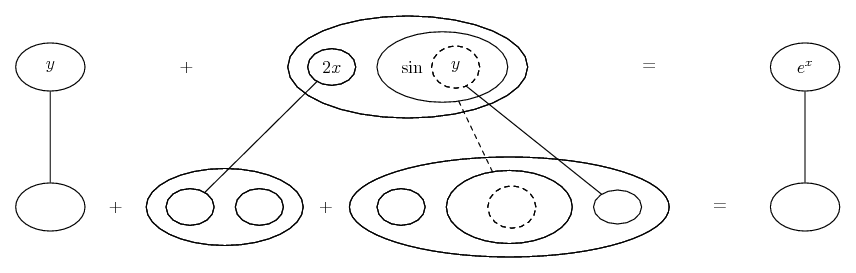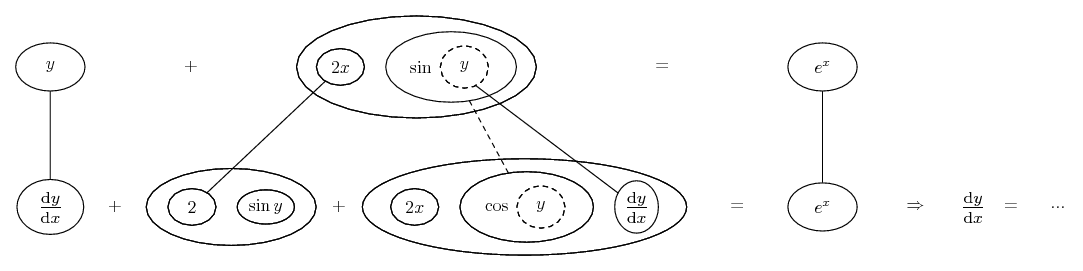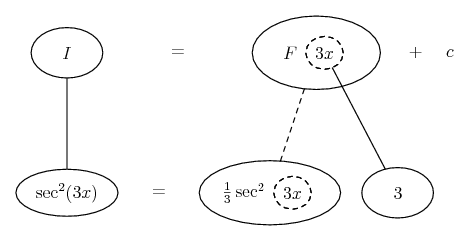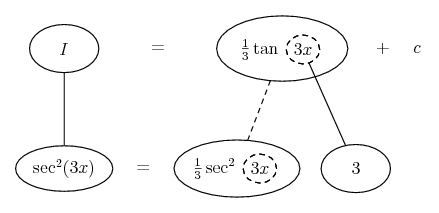# Thread: A couple of basic calculus problems

1. ## A couple of basic calculus problems

Hello,

Could I get a hint about how to deal with a couple of calculus problems:

1) The integral of Sec^2(3x)

2) The derivative of y + 2xSin(y) = e^x (i.e. dy/dx)

2. Just in case a picture helps...... where (key in spoiler)...

Spoiler:... is the chain rule, here embedded in the legs-uncrossed version of...... the product rule. Straight continuous lines differentiate downwards (integrate up) with respect to x, and the straight dashed line similarly but with respect to the dashed balloon expression (which is the inner function of the composite and hence subject to the chain rule).

Spoiler:And here, to integrate and therefore travelling up instead of down,... you're meant to integrate sec^2 as a standard integral (but see here).

Spoiler:__________________________________________

Don't integrate - balloontegrate!

Balloon Calculus; standard integrals, derivatives and methods

Balloon Calculus Drawing with LaTeX and Asymptote!

3.Originally Posted by DangerousDaveHello,

Could I get a hint about how to deal with a couple of calculus problems:

1) The integral of Sec^2(3x)
You are expected to know that the derivative of tan(x) is sec^2(x).

2) The derivative of y + 2xSin(y) = e^x (i.e. dy/dx)
Do you know "implicit differentiation"? What is the derivative of sin(y) with respect to x? What is the derivative of 2x sin(y) with respect to x?

basic, calculus, couple, problems